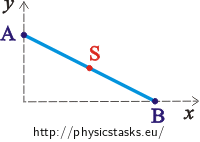## Sliding of a line segment I

Line segment AB of constant length l is moving in a way that its endpoints are sliding on the y axis or x axis of the orthogonal coordinate system. (The picture shows the situation in the 1st quadrant.)Determine the trajectory of the centre S of the line segment AB.

• #### Hint 1

Write down the coordinates of the points A, B. Are they somehow connected to the length l of the line segment AB?

• #### Hint 2

Write the coordinates of the point S. We are interested in the motion of this point - thus try to write the coordinates of the points A, B using the coordinates of the point S.

• #### Hint 3

We are interested in the movement of the centre S, that’s why we want to have its coordinates in the relation (1). Substitute thus for xy using the expressions with xs and ys and modify.

Which curve is the final relation describing?

• #### Complete solution

We express the coordinates of the points A, B:

A=[0;y],

B=[x;0].

We mark coordinates of the centre of the line segment: $$S = [x_{s};y_{s}]$$ and express $$S = [x_{s};y_{s}]=[\frac{x}{2};\frac{y}{2}].$$

Thus we can determine x and y:

$x_{s}=\frac{x}{2}\Rightarrow x = 2x_{s},$

$y_{s}=\frac{y}{2}\Rightarrow y = 2y_{s}.$

The length of the line segment can be expressed using Pythagoras theorem so we can substitute for x, y:

$l^{2}=x^{2}+y^{2}=((2x_{s})^{2}+(2y_{s})^{2})=4(x_{s}^{2}+y_{s}^{2})\,.$

After modifying we get:

$(\frac{l}{2})^{2}=x_{s}^{2}+y_{s}^{2}\,,$

which is the equation of the circle.

Thus the trajectory of the movement of the point S is a circle. (If the points A and B move only on the part of the coordinate axes which border the 1st quadrant, as shown in the picture, it is only one quarter of the circle.)

Notes:

1) We can use this principle to construct a circle: Draw two perpendicular lines on a sheet of paper. Then take a slip of paper (its length is the diameter of the future circle) and mark its centre. Place the slip of paper so that it’s endpoints lay each on a different line. When moving the endpoints on the lines, the centre of the slip of paper will create a circle.

2) If you take two not perpendicular but divergent lines at the beginning, you will get an ellipse by this method. You can also get an ellipse when the lines are perpendicular but when you choose a different point than the centre one.